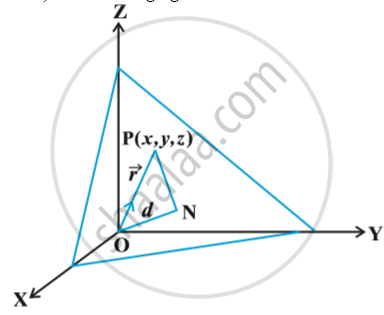# Plane - Equation of a Plane in Normal Form

#### notes

Consider a plane whose perpendicular distance from  the origin is d (d ≠ 0). in following fig.If vec (ON) is the normal from the origin to the plane, and hat n  is the unit normal vector along vec (ON). Then vec (ON) = d . hat n . Let P be any point on the plane. Therefore , vec (NP) is perpendicular to vec (ON).
Therefore, vec (NP) . vec (ON) = 0  ...(1)
Let vec r be the position vector of the point P, then vec (NP) = vec r - d . hat n     (as vec (ON) + vec (NP) = vec (OP))
Therefore, (1) becomes
(vec r - d . hat n) . d hat n = 0
or (vec r - d.hat n). hat n =0  (d ≠ 0)
or vec r . hat n - d  hat n . hat n = 0
i.e., vec r .  hat n = d        (as hat n . hat n = 1)          ...(2)
This is the vector form of the equation of the plane.

Cartesian form
Equation (2) gives the vector equation of a plane, where hat n is the unit vector normal to the plane. Let P(x, y, z) be any point on the plane.  Then
vec (OP) = vec r = x hat i + y hat j + z hat k
Let l, m, n be the direction cosines of hat n . Then
hat n = l hat i + m hat j + n hat k
Therefore, (2) gives
(x hat i + y hat j + z hat k) . (l hat i + m hat j + n hat k) = d
i.e. lx + my + nz = d                             ... (3)

If you would like to contribute notes or other learning material, please submit them using the button below.